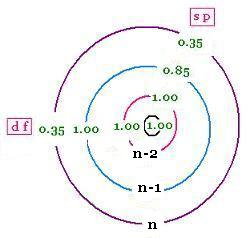Fundamentals of
Quantum Mechanics

Contents

# Slater's rules for effective nuclear charge

### 1. Slater's Rules, the steps

Slater Type Orbitals (STO) are an approximation and do not represent the realistic orbitals. This oversimplified model is found according to the semi-empirical results.

In the STO, the nuclear charge Z and the principal quantum number n are replaced by the effective nuclear charge Z*, and the effective principal quantum number n* respectively; in order to fit the two following formula for the radial function and energy of any electron in any atom:

R(r) = Nr rn* - 1 exp {- (Z*/n*) r}
Nr is the normalization constant for different orbitals.
E = - (Z*/n*)2 (13.6 eV)

R(r) = Nr rn*-1 exp {- (Z*/n*) r}
Nr is the normalization constant for different orbitals
E = - (Z*/n*)2 (13.6 eV)Here are the Slater's rules to calculate an approximate value for the effective nuclear charge "felt" by an electron in a particular orbital of an atom.

1 - Write the electronic configuration for the atom using the following grouping, called Slater electron configuration:

(1s) (2s,2p) (3s,3p) (3d) (4s,4p) (4d) (4f) (5s,5p) ... ,

2 - Any electron to the right of the considered electron of interest do not contribute to shielding,

3 - All other electrons in the same group of the considered electron shield by 0.35 nuclear charge units each; except for 1s, the screening is reduced to 0.30,

4 - If the considered electron is an s or p electron in the shell of principal quantum number n, all electrons with one less value n-1 shield by 0.85 units of nuclear charge each; and all electrons with two less values n-2 shield by 1.00 units. The n-3, n-4, ... shield by 1.00 units each as well.

5 - If the considered electron is an d or f electron: all electrons to the left shield by 1.00 units of nuclear charge each.

6 - Sum all the shielding amounts for each group. To obtain the effective charge for each group, subtract from the nuclear charge value the corresponding shielding.

7 - For larger principal quantum numbers, n is corrected as follows:
n = 1, 2, 3, 4, 5, 6
n* = 1, 2, 3, 3.7, 4.0, 4.2

### 2. Example:

Ruthenium atom: Ru: Z = 44

(1s2) (2s2 2p6) (3s2 3p6) (3d10) (4s2 4p6) (4d7) (5s1)

S(1s2) = 1 * 0.35 = 0.35
S(2s2 2p6) = 7 * 0.35 + 2 * 0.85 = 4.15
S(3s2 3p6) = 7 * 0.35 + 8 * 0.85 + 2 * 1.00 = 11.25
S(3d10) = 9 * 0.35 + 8 * 1.00 + 8 * 1.00 + 2 * 1.00 = 21.15
S(4s2 4p6) = 7 * 0.35 + 18 * 0.85 + 8 * 1.00 + 2 * 1.00 = 27.75
S(4d7) = 6 * 0.35 + 8 * 1.00 + 18 * 1.00 + 8 * 1.0 + 2 * 1.00 = 38.1
S(5s1) = 15 * 0.85 + 18 * 1.00 + 8 * 1.00 + 2 * 1.00 = 40.75

Zeff(electronic state) = Z - S(electronic state)

Zeff(1s2) = 44 - 0.35 = 43.7
Zeff(2s2 2p6) = 44 - 4.15 = 39.85
Zeff(3s2 3p6) = 44 - 11.25 = 32.75
Zeff(3d10) = 44 - 21.15 = 22.85
Zeff(4s2 4p6) = 44 - 27.75 = 16.25
Zeff(4d7) = 44 - 38.1 = 5.9
Zeff(5s1) = 44 - 40.75 = 3.25

E (4s2 4p6) = - (16.25/3.7)2 (13.6 eV) = - 262.33 eV

### 3. Z effective calculator:

Zeff Calculator

 Enter an atom's symbol Get its effective nuclear charge
##### Results:

 chimie labs | Physics and Measurements | Probability & Statistics | Combinatorics - Probability | Chimie | Optics | contact |